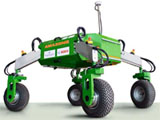## Biological Systems Engineering## Biological Systems Engineering: Papers and Publications

#### Date of this Version

2-1992

Published in JOURNAL OF IRRIGATION AND DRAINAGE ENGINEERING 118:1 (January/February 1992), pp. 104-112.

#### Abstract

A laboratory study is conducted to measure Darcy-Weisbach roughness coefficients for selected gravel and cobble materials. Varying rates of flow are introduced into a flume in which a given size class of gravel or cobble material is securely attached. Roughness coefficients are calculated from measurements of discharge rate and flow velocity. The laboratory data are used to develop regression equations for relating roughness coefficients to surface cover and Reynolds number. The regression relations, which are developed for values of the Reynolds number from approximately 500 to 16,000, are tested using hydraulic data collected on surfaces containing a distribution of size classes. Close agreement between predicted and measured Darcy-Weisbach roughness coefficients is obtained by adding the roughness contributions of individual size classes. Equations are also presented for estimating surface cover from measurements of gravel and cobble mass. Accurate prediction of roughness coefficients for gravel and cobble surfaces will improve our ability to understand and properly model upland flow hydraulics.

COinS